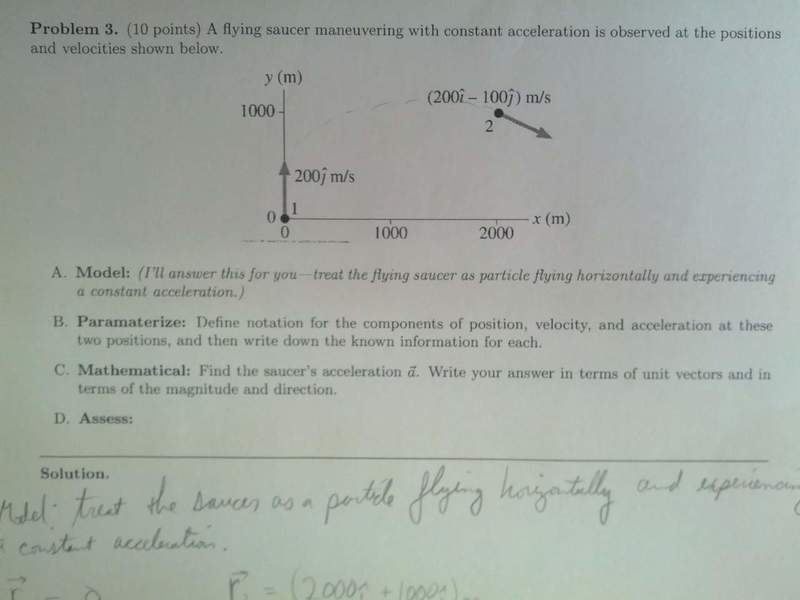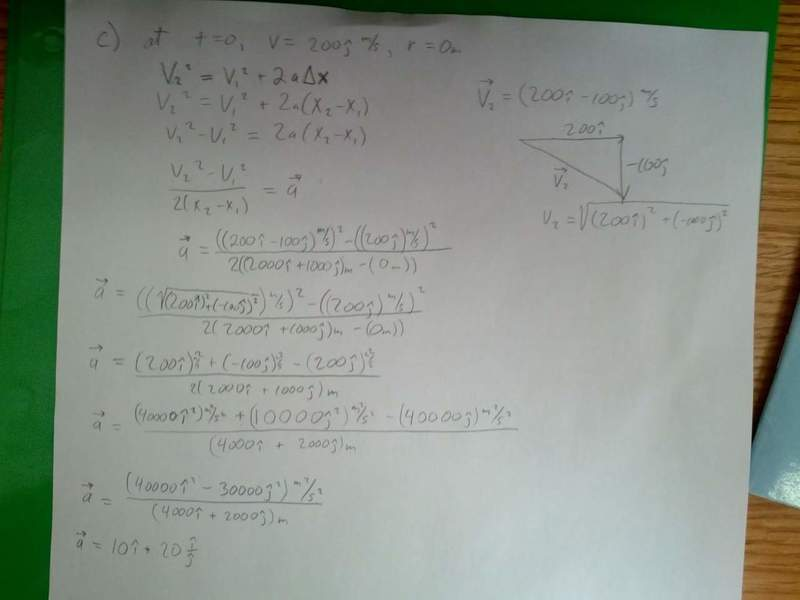# Easy-acceleration with unit vectors

• PEZenfuego
In summary, the conversation discusses kinematic equations for components of displacement, velocity, and acceleration. The speaker mentions two sets of kinematic equations for each component and asks for the saucer's acceleration in unit vectors and in terms of magnitude and direction. The other person clarifies that the given equations are valid for each component and asks for the results of the calculations.

## Homework Statementr1=(0m)
r2=(2000i + 1000j)m
v1=(200j)m/s
v2=(200i-100j)m/s

## Homework Equations

tantheta=vy/vx

v2^2=v1^2+2ax
v2=v1+at
x2=x1+v1t+1/2at^2

## The Attempt at a SolutionThank you for any help

So what do we have to find??

find the saucer's acceleration in unit vectors and in terms of magnitude and direction.

PEZenfuego said:

## Homework Statement

View attachment 50618

r1=(0m)
r2=(2000i + 1000j)m
v1=(200j)m/s
v2=(200i-100j)m/s

## Homework Equations

tantheta=vy/vx

v2^2=v1^2+2ax
v2=v1+at
x2=x1+v1t+1/2at^2

## The Attempt at a Solution

View attachment 50617

Thank you for any help
Are those kinematic equations for components of vectors, or for vectors themselves?

SammyS said:
Are those kinematic equations for components of vectors, or for vectors themselves?

If you click the first picture, you will have an understanding equal to mine. I'm sorry.

PEZenfuego said:
If you click the first picture, you will have an understanding equal to mine. I'm sorry.
Sorry for the slow response.

I should have been more direct with my earlier response.

The set of kinematic equations you have
(v2)2=(v1)2+2ax
(v2)=(v1)+at
x2=x1+(v1)t+1/2at2
are valid for each component of displacement, velocity, and acceleration.

So you have two such sets of kinematic equations:
(v2)x2 = (v1)x2 + 2axx
(v2)x = (v1)x + axt
x2 = x1 + (v1)xt + 1/2axt2

(v2)y2 = (v1)y2 + 2ayy
(v2)y = (v1)y + ayt
y2 = y1 + (v1)yt + 1/2ayt2

SammyS said:
Sorry for the slow response.

I should have been more direct with my earlier response.

The set of kinematic equations you have
(v2)2=(v1)2+2ax
(v2)=(v1)+at
x2=x1+(v1)t+1/2at2
are valid for each component of displacement, velocity, and acceleration.

So you have two such sets of kinematic equations:
(v2)x2 = (v1)x2 + 2axx
(v2)x = (v1)x + axt
x2 = x1 + (v1)xt + 1/2axt2

(v2)y2 = (v1)y2 + 2ayy
(v2)y = (v1)y + ayt
y2 = y1 + (v1)yt + 1/2ayt2

It makes sense now. thank you.

PEZenfuego said:
It makes sense now. thank you.
What did you get for answers?